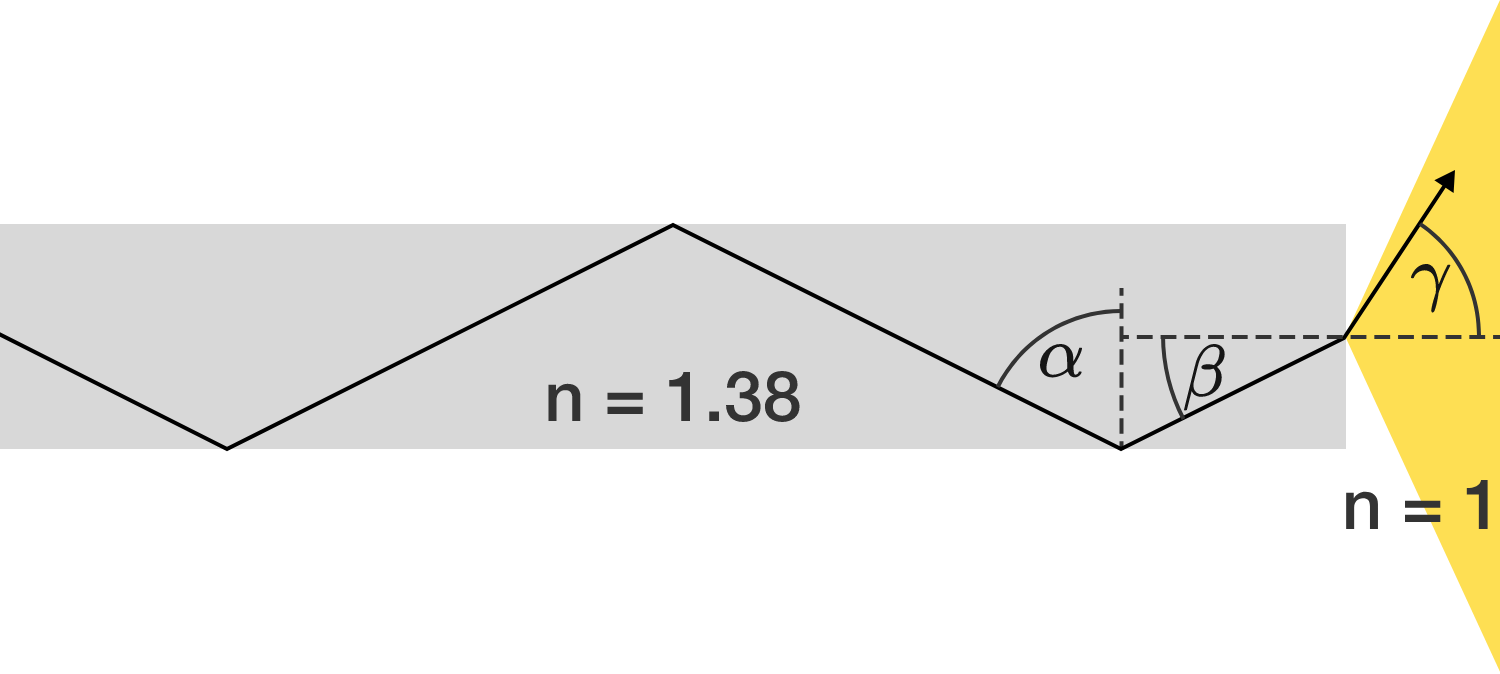# Optical fiber cable

Glass-fiber-guided light beams are continuously reflected at the interface between glass and air. How large is the maximum angle $\gamma$ (half opening angle) of the exiting light at the end of the glass fiber, in degrees, to the nearest integer?

The glass has a refractive index of $n = 1.38,$ while the air has an index of $n = 1.$×